Intermediate Geometry : How to find the angle for a percentage of a circle

Example Questions

← Previous 1

Example Question #1 : How To Find The Angle For A Percentage Of A Circle

How many degrees are in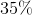of a circle?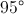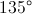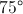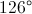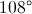Explanation:

There are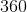degrees in a circleso the equation to solve becomes a simple percentage problem: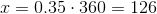Example Question #2 : How To Find The Angle For A Percentage Of A Circle

A sector contains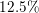of a circle.  What is the measure of the central angle of the sector?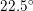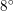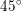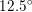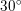Explanation:

An entire circle is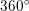. A sector that isof the circle therefore has a central angle that isof.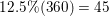Therefore, our central angle isExample Question #3 : How To Find The Angle For A Percentage Of A Circle

If you have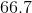percent of a circle, what is the angle, in degrees, that creates that region?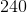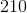Explanation:

A full circle has 360 degrees, which means that 100% of the circle is 360 degrees.

Now you need to convert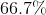into a decimal.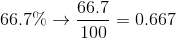If you multiply 360 by 0.667, you get the degree measure that corresponds to the percentage, which is 240.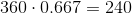Example Question #4 : How To Find The Angle For A Percentage Of A Circle

If you haveof a circle, what is the angle, in degrees, that creates that region?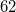Explanation:

A full circle has 360 degrees, which means that 100% of the circle is 360 degrees.

First convertinto a decimal.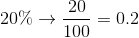If you multiply 360 by 0.20, you get the degree measure that corresponds to the percentage, which is 72.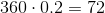Example Question #5 : How To Find The Angle For A Percentage Of A Circle

If you have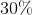of a circle, what is the angle, in degrees, that creates that region?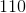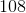Explanation:

A full circle has 360 degrees, which means that 100% of the circle is 360 degrees.

In order to start this problem we need to convert the percent into a decimal.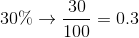If you multiply 360 by 0.30, you get the degree measure that corresponds to the percentage, which is 108.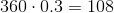Example Question #6 : How To Find The Angle For A Percentage Of A Circle

If you haveof a circle, what is the angle, in degrees, that creates that region?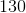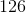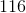Explanation:

A full circle has 360 degrees, which means that 100% of the circle is 360 degrees.

First convert the percent to decimal.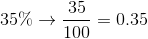Now if you multiply 360 by 0.35, you get the degree measure that corresponds to the percentage, which is 126.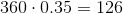Example Question #7 : How To Find The Angle For A Percentage Of A Circle

If you have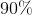of a circle, what is the angle, in degrees, that creates that region?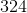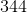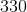Explanation:

A full circle has 360 degrees, which means that 100% of the circle is 360 degrees.

First convert the percentage into a decimal.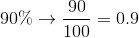If you multiply 360 by 0.90, you get the degree measure that corresponds to the percentage, which is 324.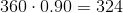Example Question #8 : How To Find The Angle For A Percentage Of A Circle

If you have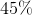of a circle, what is the angle, in degrees, that creates that region?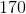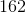Explanation:

A full circle has 360 degrees, which means that 100% of the circle is 360 degrees.

First we need to convert the percentage into a decimal.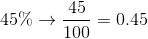If you multiply 360 by 0.45, you get the degree measure that corresponds to the percentage, which is 162.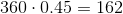Example Question #9 : How To Find The Angle For A Percentage Of A Circle

If you have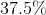of a circle, what is the angle, in degrees, that creates that region?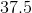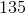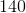Explanation:

A full circle has 360 degrees, which means that 100% of the circle is 360 degrees.

In order to solve this problem we first need to convert the percentage into a decimal.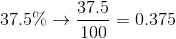If you multiply 360 by 0.375, you get the degree measure that corresponds to the percentage, which is 135.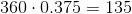Example Question #10 : How To Find The Angle For A Percentage Of A Circle

If you haveof a circle, what is the angle, in degrees, that creates that region?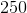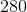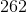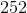Explanation:

A full circle has 360 degrees, which means that 100% of the circle is 360 degrees.

First we need to convert the percentage into a decimal.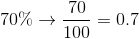If you multiply 360 by 0.70, you get the degree measure that corresponds to the percentage, which is 252.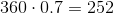← Previous 1